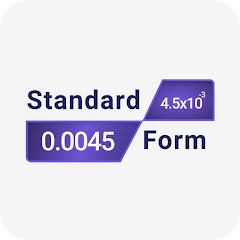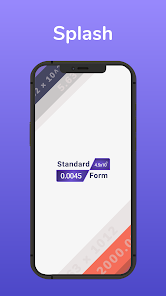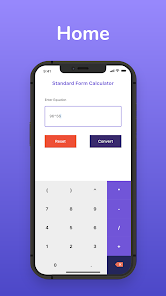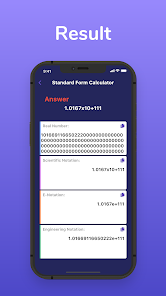# Standard Form Calculator

100+EveryoneThe standard form converter converts the given number to:

Scientific notation
Real number
E-Notation, and
Engineering notation
Standard notation converter accepts values in decimal form and converts the input to standard form in a heartbeat.

Keep on reading if you are interested in standard form definition, how to find standard form without standard form generator, and a few examples of standard or scientific notation.
How does it work?
Enter the number or simple mathematical expression.
Click the Convert button.
The standard form calculator will convert the number into four different notations.
Do you know what does standard form mean?

Standard Form is the term in mathematics that is used for converting long or complex numbers into the simplest form for reducing the difficulty to read the number.
We can say that standard form is a method of writing very large and very small numbers in the power of ten. For instance, the same number 98400, when written in standard form, will become 9.84 x 104.

The power often depends on the number, if it is too small such as 0.00003, then in standard form, we will move the decimal point to the right direction and its standard form will be 3 x10-5.

Converting a small value into standard form involves moving the decimal point to the right, the power of ten will be negative. On the other hand, while dealing with very large numbers, the power of ten will be positive.

This free standard form app is very beneficial for every student while converting numbers in standard form.
Just install this amazing app on your smartphone and start doing calculations.
Updated on
Jan 10, 2022

## Data safety

Safety starts with understanding how developers collect and share your data. Data privacy and security practices may vary based on your use, region, and age. The developer provided this information and may update it over time.No data shared with third parties